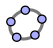Back to Function Diagrams

# Function Diagram Applets

by Henri PicciottoDynamic: with graph | composition | linear | 2 linear | magnification | mystery | y=1/x
Static (to copy-paste): Diagram | Composition | Iteration

For an introduction to function diagrams and their use in secondary math education, see Function Diagrams.

To download packets of dynamic geometry files (GeoGebra or Cabri) and TI calculator programs, go to Function Diagrams: Electronic Tools.

Here are links to applets I created to help in the study of functions through animated and manipulable function diagrams. I created them using GeoGebra, so if you want to use these applets offline, or make changes to them, all you need to do is download this packet of GeoGebra files.

## General instructions

You can explore by dragging free points (usually larger hollow points in the figures). In particular, moving the '1' on the x number line allows you to change the scale.

There is a button at the top right of the graphics/ panes to restore the figure to its initial state.

In some cases, you can use the input bar at the bottom of the applet to redefine the function under study.

If there is a 'play' ▷ button (for animation), it will be at the bottom-left of the graphics/ pane. Clicking 'pause' ‖ will stop the animation.

## The Applets

### Dynamic

The notes below are intended to suggest one possible sequence of explorations and to provide a table of contents of the available applets.

An applet which allows you to simultaneously look at the function diagram and the Cartesian representation of a function. It can be used to introduce function diagrams.

An applet which allows you to look at the composition of two functions. One possible goal is to define g so that it is the inverse function of f. The resulting figure makes clear that in that case g's function diagram is the mirror image of f's.

An applet to dynamically find the linear function that corresponds to a given focus as you move the focus. An interesting discussion may be started by the question: how does the computer calculate the m and the b given the position of the focus?

Another one that includes the foci for two linear functions, and can be manipulated in the same way. It allows you to find the solution of a system of simultaneous linear equations in two variables by finding the common in-out line. It can also be used to find inverse linear functions by looking (manually) for foci in symmetric positions. And it makes a good background to a discussion of families of linear functions: where would the focus be for functions with the same m? with the same b?

An applet to explore magnification, or how the rate of change shows up in a function diagram. You can control the value of Δx, and see how it affects Δy/Δx.

Name that function! One applet that contains nine different mystery functions. To evaluate the validity of the students' guesses about what functions are represented, the animation can be stopped, and the x can be dragged manually. (Suggested level: Precalculus, but would work at the end of some Algebra 2's or at the beginning of a Calculus course. To change the functions to ones more appropriate to your situation, download the GeoGebra file, and edit the "images" list.)

Two applets about the function diagram for y=1/x. One shows that the in-out lines are all tangent to an ellipse, the other is useful in proving this result.

### Static

These applets are intended to create diagrams you can copy-paste into word processors or presentation software.

A static diagram for any function.

Linked diagrams to illustrate composition of two functions.

Linked diagrams to illustrate iteration of a linear function.Related pages on this site:
Function diagrams overview
Electronic tools for function diagrams
Function diagram PDFs
Applets Directory